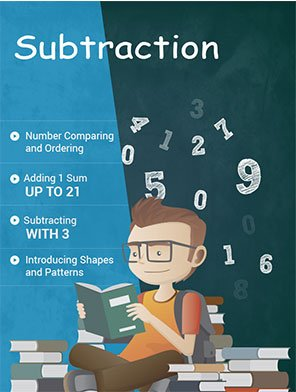\$7.00

## MATH SUBTRACTION WORKSHEETS

Our math subtraction worksheets first allow students to write numbers in descending order. Students will then begin subtraction exercises, subtracting 1 from all numbers up to 20. Similarly, they will continue subtracting 2 to 9 and onward with each exercise subtracting increasing variety and complexity to make them efficient in subtraction concepts. Subtraction problems of 24 units from this section include subtracting numbers in horizontal and vertical form, and subtracting 4 to 1 digit numbers from 4 digit numbers, subtracting three numbers, and subtracting numbers ending at 0 or 0 at the middle.

Worksheet # Topics/Curriculum # of Pages 2pg Sample Add to cart
Unit-2 Subtracting with 2 8 pages\$1.00

Unit-3 Subtracting with 3 8 pages\$1.00

Unit-4 Subtracting with 4 8 pages\$1.00

Unit-5 Subtracting with 5 8 pages\$1.00

Unit-6 Subtracting with 6 and 7 8 pages\$1.00

Unit-7 Subtracting with 8 and 9 8 pages\$1.00

Unit-8 Subtracting 0-9 Randomly 8 pages\$1.00

Unit-9 Subtracting 0-10 from Numbers up to 30 8 pages\$1.00

Unit-10 Subtracting 0-12 from Numbers up to 30 8 pages\$1.00

Unit-11 Subtracting 0-14 from Numbers up to 30 8 pages\$1.00

Unit-12 Subtracting 0-16 from Numbers up to 30 8 pages\$1.00

Unit-13 Subtracting 0-17 from Numbers up to 30 8 pages\$1.00

Unit-14 Subtracting 0-18 from Numbers up to 30 8 pages\$1.00

Unit-15 Subtracting 0-19 from Numbers up to 30 8 pages\$1.00

Unit-16 Subtracting 0-20 from Numbers up to 30 8 pages\$1.00

Unit-17 Subtracting 1 Digit Numbers in Vertical Form 8 pages\$1.00

Unit-18 Subtracting 1 or 2 Digit Numbers from 2 Digit Numbers 8 pages\$1.00

Unit-19 Subtracting 2 Digit Numbers from 2 Digit Numbers 8 pages\$1.00

Unit-20 Subtracting Tens from Hundreds 8 pages\$1.00

Unit-21 Subtracting 3 Digit Numbers from 3 Digit Numbers 8 pages\$1.00

Unit-22 Subtracting 2 or 3 Digit Numbers from 3 Digit Numbers 8 pages\$1.00

Unit-23 Subtracting Numbers Ending in 0 or with a 0 in the Middle 8 pages\$1.00

Unit-24 More Problems on Subtraction 8 pages\$1.00